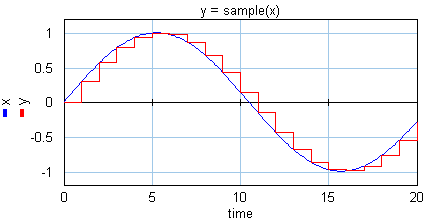﻿ 20-sim webhelp > Language Reference > Functions > Discrete > sample

# sample

## Syntax

y = sample(x,init);

## Description

The sample function implements a sample and hold function operating at a specified sampling rate. It provides a mechanism for discretizing a continuous input signal. The initial value of y is equal to the value of init. This initial value is optional. If no value is entered, a default initial value of 0 is used.## Examples

x = sin(time*0.3);

y = sample(x);

z = previous(y);

u = hold(z);

## Limitations

 • x and y must be scalars. x represents a continuous signal and y represents a discrete signal.
 • y will become a discrete signals. 20-sim will automatically detect the existence of discrete signals. Each chain of discrete signals will be assigned a specific sample time. You can set this sample time to any desired value in the Simulator (choose Properties, Simulation and Discrete System).